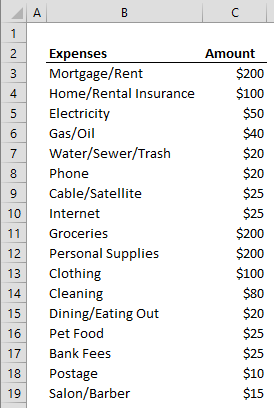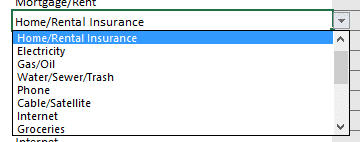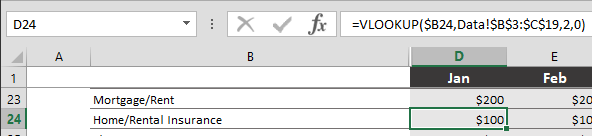You may have built various data models, created complex calculators, and published monthly reports, or maybe you’re just a complete beginner when it comes to spreadsheets. Either way, Excel’s plethora of formulas, tables, shortcuts, and layout make it the perfect tool for doing your personal finances too!

We’ve compiled a list of formulas we believe are most useful when doing personal finances in Excel. We hope you take it as a refresher or find it a good place to start. You can download our sample workbook below.

# SUM

Instead of selecting individual cells and entering ‘+’ in between, use the SUM function to add up an entire range of values. When doing your personal finances, there are two main reasons why you should choose the SUM function instead of regular addition operator (+).

The first advantage of the SUM function is that you don't need to write every cell one by one. You can select all adjacent cells at once and the SUM function add all values in that range.

Another advantage of the SUM function is that it adds all numerical values, meaning that it avoids all non-numeric values and only adds numerical values (using a plus sign (+) returns #VALUE! error instead).

=SUM(number1,[number2],...)# SUBTOTAL

The SUBTOTAL function is the Swiss Army Knife of the functions. It literally can do what 11 functions can, from calculating averages, to finding variance of numbers. You can define its purpose by placing a number from 1 to 11 to its first argument. We use 9 as our first argument, which means SUM (So, the function will work as the SUM function). However, this is not why we decided to include this formula.

The SUBTOTAL function can avoid other SUBTOTAL functions when running calculations. This behavior becomes handy for when you need to show subtotals, as the name suggests.

=SUBTOTAL(function_num,ref1,[ref2],...)

Let's see how this works on an example.The cell D7 contains a SUBTOTAL function with SUM (9) ability and the range D8:D19 is to be added. However, we already have two sets of totals at rows 8 and 15, which would return double the value in normal circumstances. When using SUBTOTAL in both of those cells, we’re not counting totals multiple times. Essentially, the SUBTOTAL in D7 avoids SUBTOTALS in D8 and D15 when calculating.

# VLOOKUP

The famous VLOOKUP formula can vertically look up data within tables. This formula will be most useful for dynamically calculating budgeting tools, so you won’t have to worry about formatting or other functions.

The VLOOKUP uses the first column of your data to search for the lookup value. After finding the lookup value in a row, it returns the value specified in the column number, in table array. The very last parameter determines whether the formula should look for an exact or approximate string (range lookup).

=VLOOKUP(Lookup_value, table_array, col_index_num, [range_lookup])

For example, begin by creating a list of your items and their base amounts.Make a drop-down list by selecting the entire list and go to Data Validation.Finally use the VLOOKUP function for monthly costs.Before copying your formula to other months, make sure that the search column of the first argument and the whole data range are absolute references. The dollar sign (\$) indicates that a row or column reference that comes before it is absolute. For instance, \$B24 means column B is absolute, but row number is still relative (will be updated when copied).

For more detailed information see, HOW-TO VLOOKUP

# AutoSum

The AutoSum is not a function, but a useful feature that can help you create budgeting applications faster. AutoSum menu can be found under the FORMULAS ribbon. For operations like sum, average, count, maximum, or minimum of a set of values, this is one of the easiest methods.Selecting one of these options will insert the corresponding function into the selected cell. AutoSum is a smart feature as it can predict the range you want to use in the function, and automatically place the function you choose.

Begin by selecting your data and then make your selection or press the shortcut for that action. For example, you can press Alt + = to add SUM functions below your data. Alternatively, you can choose any function you want under menu option.